Courses

# Point Load Civil Engineering (CE) Notes | EduRev

## Civil Engineering (CE) : Point Load Civil Engineering (CE) Notes | EduRev

The document Point Load Civil Engineering (CE) Notes | EduRev is a part of the Civil Engineering (CE) Course Advanced Solid Mechanics - Notes, Videos, MCQs & PPTs.
All you need of Civil Engineering (CE) at this link: Civil Engineering (CE)

The first boundary value problem that we study for the beam on elastic foundation is when it is subjected to a point load at its mid span as shown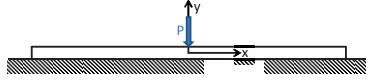Figure 11.2: Schematic of a long beam on elastic foundation subjected to concentrated load at mid span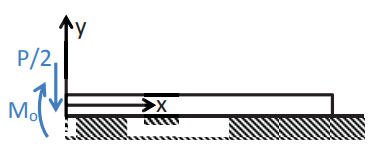Figure 11.3: Free body diagram of half the section of long beam on elastic foundation subjected to concentrated load

in figure 11.2. The origin of the coordinate system is assumed to coincide with the point of application of the load. The beam length is assumed to be large enough compared to its lateral dimension that it can be considered to be infinitely long. (We shall quantify what length could be considered as infinitely long after we obtain the solution.)

To obtain the solution we section the beam at x = 0, the point of application of the concentrated load, as shown in figure 11.3. Since, there is a concentrated force acting at x = 0, the shear force would be discontinuous at x = 0 and hence the fourth derivative of the deflection, ∆ does not exist. Consequently, the governing equation (11.9) is valid only in the domain x > 0 and x < 0 and not at x = 0. Therefore, we segment the beam at x = 0 and solve (11.9) on each of the segments. Then, we ensure, the differentiability of the second order derivative of deflection so that the third order derivative exist. This is required to ensure the existence of shear force at x = 0.

We also expect the deflection to be symmetric about x = 0 that is, ∆(x) = ∆(−x) and therefore the slope of the deflection should be zero at x = 0,

i.e.,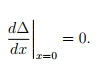(11.12)

By sectioning the beam at x = 0 we find the bending moment and shear force at this location. Using equation (11.2) we find that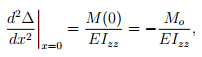(11.13)

where Mo is the bending moment at x = 0, acting as shown in the figure 11.3 and the negative sign is to account for the fact that it is hogging. Since, shear force should exist, the continuity of the bending moment and d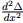has to be ensured. Therefore the value of bending moment at both the segments of the beam should be the same.
Substituting equation (11.2) in (11.3) and assuming the beam to be homogeneous and prismatic, we obtain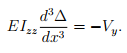(11.14)

Since, there is a concentrated force at x = 0, Vy(0+) = −Vy(0−) and the equilibrium of an infinitesimal element centered about x = 0 requires that Vy(0+) − Vy(0−) = P. Hence, Vy(0+) = P/2 and Vy(0−) = −P/2. Thus, from equation (11.14) we obtain,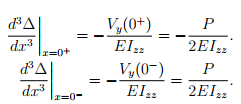(11.16)

Further, we require that

∆ → 0, as x → ±∞,                   (11.17)

since, we expect the effect of the load would be felt only in its vicinity. To obtain the solution, we first focus on the right half of the beam wherein x > 0. Then, the requirement (11.17) implies that the constants C3 and Cin the general solution (11.10) has to be zero; otherwise ∆ → ∞ as x →∞. Next, the condition (11.12) requires that C= C2 = C0. Finally, the equation (11.15) tells us that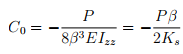(11.18)

where to obtain the last equality we have made use of (11.8). Thus, in the domain, x > 0,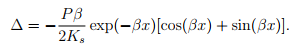(11.19)

Now, we consider the left half of the beam, i.e., x < 0. The requirement (11.17) implies that the constants Cand C2 in the general solution (11.10) has to be zero. Next, the condition (11.12) requires that C3 = −C4 = C5. Finally, the equation (11.16) tells us that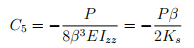(11.20)

Thus, the distribution of the reaction from the foundation along the axis of the beam given in (11.1) evaluates to: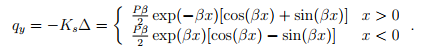(11.22)

The variation of the bending moment along the axis of the beam obtained from (11.2) is: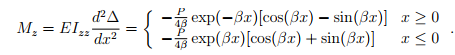(11.23)

The shear force variation along the axis of the beam computed using (11.14) is: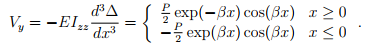(11.24)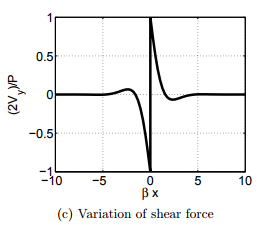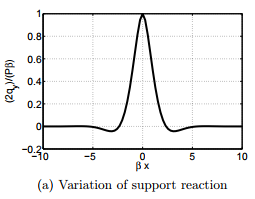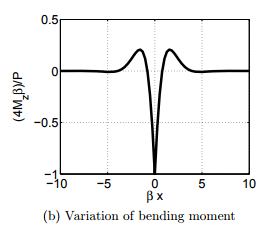Figure 11.4: Variation of support reaction, bending moment and shear force along the axis of the beam on elastic foundation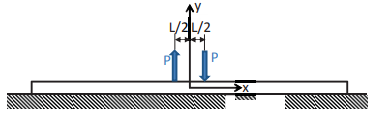Figure 11.5: Schematic of a long beam on elastic foundation subjected to concentrated moment at mid span

In figure 11.4 we plot the variation of the support reaction, bending moment and shear force along the axis of the beam. It can be seen from the figure that though the beam is assumed to be infinitely long the reaction force, bending moment and shear forces all tend to zero for βx > 5. Hence, a beam may be considered as long if its length is greater than, 5/β. It can also be seen from the figure that the maximum deflection, support reaction, bending moment and shear occurs at z = 0 and these values are,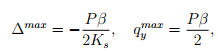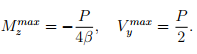(11.25)

It can be seen from figure 11.4a that the support reaction changes sign. The support reaction changes sign at a point when qy = 0, i.e., sin(βx) = − cos(βx) or at x = 3π/(4β). Since, the support reaction is proportional to the deflection, ∆, this change in sign of the support reaction also tells us that the beam will uplift at x = ±3π/(4β). Hence, the beams have to be adequately clamped to the foundation to prevent it from uplifting.

Offer running on EduRev: Apply code STAYHOME200 to get INR 200 off on our premium plan EduRev Infinity!

,

,

,

,

,

,

,

,

,

,

,

,

,

,

,

,

,

,

,

,

,

;